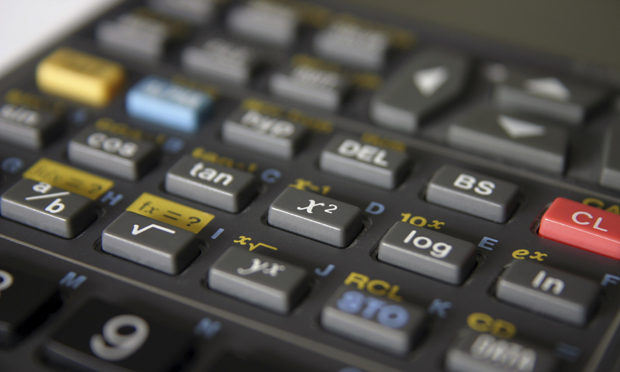Math basics for journalists: Working with averages and percentages(iStock)
By

June 15, 2014

Journalists are often thought of as being “word people,” and however true that may or may not be, even the most diligent reporter can blanche when faced with a thicket of figures. But sidestepping or downplaying numbers can be perilous.

Appropriately and accurately used, they can be the very foundation of a story — a project is over or under budget; students’ graduation rates are above or below average; prices are collapsing or spiking. It can even tell you when something is fact or folly.

Truly understanding numbers and being able to clearly convey their meaning to readers is a crucial part of being a journalist. You should have a working knowledge of arithmetic, be comfortable with statistics and be able to calculate percentages, ratios and other relationships between numbers. You should also understand what margin of error in polling is, have a basic understanding of probability and be able to work with graphs and charts. And, most important, you should be able to use this knowledge to help your readers better understand the issues at hand.

Here are a few basic concepts to keep in mind when number-crunching for a story. If you’d like to dig deeper, a good place to start is “News and Numbers: A Guide to Reporting Statistical Claims and Controversies in Health and Other Fields.”

Averages

The three mathematical concepts — “mean,” “median” and “mode” — are often lumped into the colloquial term “average.” All three measure different things, however, and using them interchangeably or in the wrong context can result in an incorrect story.

Mean: The mean is known more commonly as an average. It is calculated by dividing the sum of values in a collection by the number of values. For example, 300 + 400 + 500 = 1200. Divide this by three (the number of values we’re looking at), and the result is 400 — the mean. The mean is a good tool when all the values are relatively close in amount to show the “central tendency.” If most of the values are similar and one value is much higher or lower, the mean will not be a precise estimate of the commonality.

Median: If there is an extremely high or low value in a collection of values — say, one person with a high income in a group of low-income residents — the median is often a more accurate way to measure the central tendency of the group of numbers.

To find the median of a collection of values, sort the values in descending order. If the number of values being examined is odd (3, 11, 131, etc.), the value directly in the middle is the median. For example, the median of 50, 300, 400, 500 and 5,000 is 400 — the value in the center of the list of values.

If the collection is an even number of values, take the two values in the middle and calculate their mean by adding them together and dividing by two. For example, the median value of 300, 400, 500 and 600 is the average of the two middle values, 400 and 500: 400 + 500 = 900; 900 / 2 = 450.

Mode: Used less frequently in journalism than in statistics, the mode is more of an indicator than an accurate measurement. However, it can be helpful in determining a pattern of reoccurrence. The mode is the most reoccurring value in a collection. For example, in the numbers 300, 400, 500, 400, 200 and 400, 400 is the mode — it occurs three times in a group of six numbers.

Percentages

In news stories with raw numbers, percentages are often helpful to describe the data to readers in a meaningful way. A percentage is a fraction of a whole, or of 100. It is calculated by multiplying the numerical value by 100.

Finding a percentage: To calculate what percentage a number is of another larger number, divide the former by the latter. For example, to determine what percentage 62 among 1,567 total is: 62 / 1567 = 0.0395. Multiply this figure by 100 to get a percentage: 3.95%.

Percent change: To help readers understand the difference in a changing set of numbers, such as time or price, a percent change can be helpful. To calculate the percent change, subtract the initial value from the changed value, divide by the initial value, and then multiply that value by 100. For example, to find the percent change of a price increase from \$20 to \$35: \$35-\$20 = \$15; \$15 / \$20 = 0.75; and 0.75 x 100 = 75%.

Percent of whole: When you are describing how much one value among a collection of values contributes to the entire collection, a percentage of whole is a good place to start. To calculate a percentage of whole, add all the values in the collection. Take the particular value you are interested in and divide it by the sum of all the values. Then, multiply by 100 to find the percentage.

For example, a local news website has an investigative-reporting team. If the two-person team is paid \$2,000 a month, other salaries are \$2,000, Web services are \$1,000, and other expenses \$5,000, what percentage of the total monthly expenses are spent on investigative reporting? Total expenses are \$10,000, so \$2,000 / \$10,000 = 0.2; 0.2 x 100 = 20%.

When working with numbers, try not to let them tell the story. Instead of overwhelming your readers with a barrage of numbers, use only what is necessary to convey the essence of the story. Visual and descriptive cues help readers quickly understand content, so use rates and percentages when possible instead of raw numbers.

Tags: training, numeracy